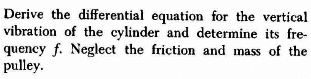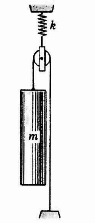# Find the frequency of the cylinder's vertical vibration

## Homework Statementw = √k/m
w = 2π/P
ƒ = 1/P

## The Attempt at a Solution

I feel like I'm so close to the answer, I think it has something to do with the fact that there's a spring and pulley in the system that is throwing my answer off. I have

√k/m = 2π/P

So,

P = 2π(√m/k) Then we take the number one and divide it by P to find frequency

1/2π(√m/k) which I get to be

ƒ = √(k/m) / 2π, which is close, but not quite. The answer is actually

ƒ = √(k/m)/(4π)

Can anyone help me understand where I'm going wrong? Thank you! :)

#### Attachments

TSny
Homework Helper
Gold Member
Can you repost the figure so that the entire figure is visible?

I'm not sure what you mean. The cylinder, pulley and spring is the entire figure. Or is the picture not visible?

TSny
Homework Helper
Gold Member
The picture is visible, but I can't tell what is at the bottom end of the string on the right side.
Edit: OK, I thought there was more to it. The string is fixed at the lower right. Sorry, I get it now.

Oh, I believe the bottom of the string is attached to a fixed end.

TSny
Homework Helper
Gold Member
Oh, I believe the bottom of the string is attached to a fixed end.
Thanks.

TSny
Homework Helper
Gold Member
If the spring stretches by an amount Δx, what is the vertical displacement Δy of the cylinder?

Would Δy = 1/2Δx?

TSny
Homework Helper
Gold Member
Yes. No, but you have the right idea.

Okay,but how exactly would the change in displacement affect the frequency? Would the displacement affect the value of K? Therefore, changing our ω?

TSny
Homework Helper
Gold Member
No, the spring constant is an inherent property of the spring. k is not affected by displacements of the spring or cylinder. I spoke too soon in post 9 where I first agreed with your answer in post 8. Do you see the correct relation between Δy of the cylinder and Δx of the spring?

Next, you might consider the situation where the cylinder is hanging at rest. What is the tension in the string? What is the force of the spring? How much is the spring stretched when the cylinder is hanging at rest?